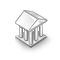Interesting script?
So post a link to it -
let others appraise it
You liked the script? Try it in the MetaTrader 5 terminal# Radix sort (The fastest numeric sort) - library for MetaTrader 5

Views:
1487
Rating:
Published:
2022.03.27 21:56
Updated:
2023.02.10 13:31

RadixSort sorts numeric data (integers or float) by considering a string of numbers where digit by digit sort starting from least significant digit position to most significant digit position.

### Time Complexity and Performance

Radix sort has linear time complexity, it operates in O(n * k) time, where:

n = the number of elements to sort
k = the maximum element width (number of bytes) of the elements to sort

The algorithm iterates over k byte places in LSD (least significant digit) order; for each byte place, it iterates over all n elements to sort them by the k-th byte.

This is a highly-optimized implementation of LSD RadixSort in MQL using radix 256 (8-bits). This could be useful for sorting huge arrays with millions of numbers.

The function is at least 3-10 times faster than MQL's built-in ArraySort() function.

The function accepts any-dimensional arrays of simple type (char, uchar, short, ushort, int, uint, long, ulong, bool, color, datetime, float, double) as a parameter. However, sorting is always applied to the first (zero) dimension.

An array is always sorted in the ascending order irrespective of the AS_SERIES flag value.

Note that, the algorithm falls back to ArraySort() if the array contains less than some threshold of items (currently 128).

Radix sort should be the default for sorting numeric data as it operates in O(n * k) time. Comparison-based sorting algorithms (like quicksort, mergesort and heapsort) run in O(n * log n) time. Thus, Radix sort is comparatively faster, the larger the array size. The downside of radix sort is that it needs more memory (temporary array) to do its work.

```//+------------------------------------------------------------------+
//+------------------------------------------------------------------+
//| Sorts the values in the first dimension of a multidimensional    |
//| numeric array in the ascending order.                            |
//|                                                                  |
//| Arguments:                                                       |
//| array[]                                                          |
//|   [in][out] : Numeric array for sorting.                         |
//|                                                                  |
//| Return value: true if successful, otherwise false.               |
//|                                                                  |
//| Note:                                                            |
//|   An array is always sorted in the ascending order irrespective  |
//|   of the AS_SERIES flag value.                                   |
//|                                                                  |
//|   The function accepts any-dimensional arrays of simple type     |
//|   (char, uchar, short, ushort, int, uint, long, ulong, bool,     |
//|   color, datetime, float, double) as a parameter.  However,      |
//|   sorting is always applied to the first (zero) dimension.       |
//|                                                                  |
//| Performance:                                                     |
//|   This is a highly-optimized implementation of LSD RadixSort     |
//|   in MQL using radix 256 (8-bits). This could be useful for      |
//|   sorting huge numeric arrays with millions of numbers.          |
//|   It is at least 3-10 times faster than built-in ArraySort().    |
//+------------------------------------------------------------------+
template<typename T>

//+------------------------------------------------------------------+
//+------------------------------------------------------------------+
//| Sorts the values in the first dimension of a multidimensional    |
//| numeric array in the ascending order.                            |
//|                                                                  |
//| Function overload for higher-dimensional numeric arrays.         |
//|                                                                  |
//| Arguments:                                                       |
//| array[]                                                          |
//|   [in][out] : Numeric array for sorting.                         |
//|                                                                  |
//| Return value: true if successful, otherwise false.               |
//+------------------------------------------------------------------+
// Sorts two-dimensional numeric arrays.
template<typename T>

// Sorts three-dimensional numeric arrays.
template<typename T>

// Sorts four-dimensional numeric arrays.
template<typename T>

//+------------------------------------------------------------------+
//+------------------------------------------------------------------+
//| Populate an array of indices[] in the order that would sort      |
//| the values of a numeric array[] in the ascending order.          |
//|                                                                  |
//| Arguments:                                                       |
//| array[]     : Array with numeric values to sort                  |
//| indices[]   : Array for sorted indices                           |
//|                                                                  |
//| Return value: true if successful, otherwise false.               |
//+------------------------------------------------------------------+
template<typename T>
bool RadixSortIndices(const T &arr[], int &indices[]);

//+------------------------------------------------------------------+
//+------------------------------------------------------------------+
//| The function sorts array[] and items[] simultaneously using      |
//| RadixSort algorithm. After sorting the items[] array is sorted   |
//| by the numeric values of array[] in the ascending order.         |
//|                                                                  |
//| Arguments:                                                       |
//| array[]     : Array with numeric keys to sort                    |
//| items[]     : Array for items to sort based on keys              |
//|                                                                  |
//| Return value: true if successful, otherwise false.               |
//+------------------------------------------------------------------+
template<typename T,typename TItem>

```

This is a benchmark script that demonstrates the speed advantage of RadixSort() over MQL's ArraySort():

```//+------------------------------------------------------------------+
//|                             https://www.mql5.com/en/users/amrali |
//+------------------------------------------------------------------+

#define SIZE 10*1000*1000  // 10 millions
int     arr1[SIZE];
int     arr2[SIZE];
//+------------------------------------------------------------------+
//|                                                                  |
//+------------------------------------------------------------------+
void OnStart()
{
//--- Prepare unsorted arrays.
for(int i = 0; i < SIZE; i++)
{
arr1[i] = MathRand();
arr2[i] = arr1[i];
}

//--- ArraySort() benchmark.
uint t1 = GetTickCount();
ArraySort(arr1);
uint ticks1 = GetTickCount() - t1;

uint t2 = GetTickCount();
uint ticks2 = GetTickCount() - t2;

//--- Display results.
PrintFormat("Sorting %d integers: ", SIZE);
PrintFormat("ArraySort() -> %u millisec", ticks1);
PrintFormat("Speed-up factor is %.1fx", (double)ticks1/ticks2);
PrintFormat("%s", ArrayCompare(arr1, arr2) ? "RadixSort: invalid sort order.": "");
}
//+------------------------------------------------------------------+

// sample output:
/*
Sorting 10000000 integers:
ArraySort() -> 953 millisec
Speed-up factor is 15.4x
*/
```

To replace MQL's ArraySort() by RadixSort() in your old mq5 or mqh files, just add these two lines at the top of the file:

```#include "RadixSort.mqh"

```Percentage of price movement per candle - Simple and Crucial

This indicator displays the percentage of price movement per candle, as an average of the latest candles.Currency Strength Meter MT5

Currency Strength Meter for MetaTrader 5 with configurable timeframe parameter, It was converted from "Currency Strength Giraia 28 pairs TRO MODIFIED" MetaTrader 4 versionAlert Crossing Three MAs

The indicator shows signals ('Arrow' objects) of the 'Moving Average' indicator crossings. The peculiarity of the indicator: if there was an intersection of 'MAs' (on bar #0), and then the intersection disappeared, the signal remains on the chartRSI_MAonRSI_Filling

'RSI' line, 'RSI' line smoothed with 'MA'. Fill areas between these two lines.